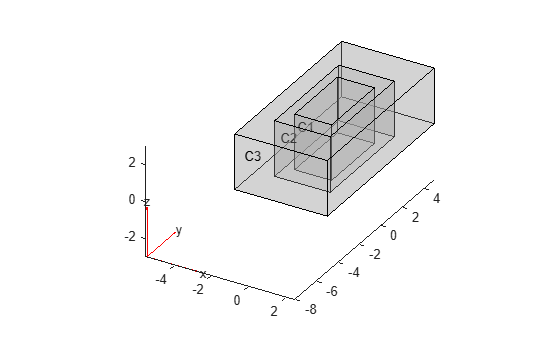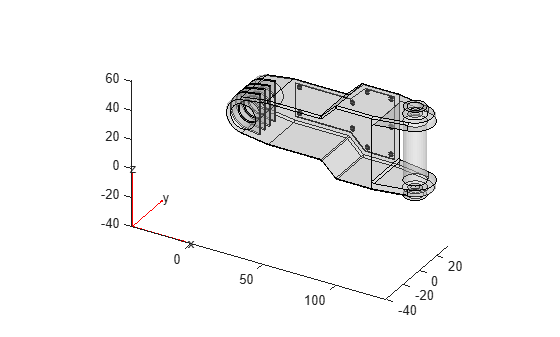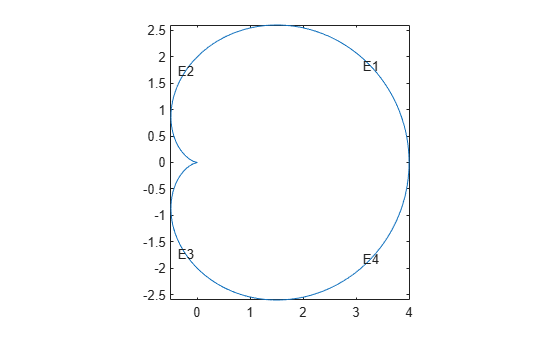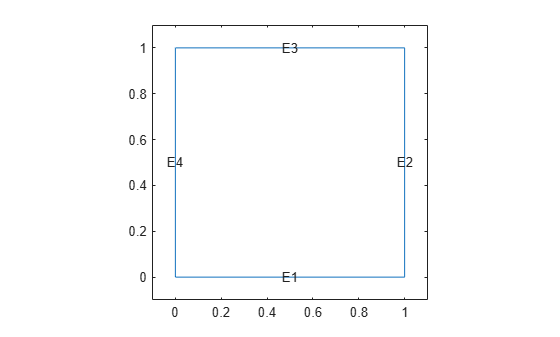# fegeometry

Geometry object for finite element analysis

Since R2023a

## Description

An `fegeometry` object contains a geometry for use in a finite element analysis with an `femodel` object.

## Creation

### Syntax

``gm = fegeometry(geometry)``
``gm = fegeometry(geometry,MaxRelativeDeviation=d)``
``gm = fegeometry(nodes,elements)``
``gm = fegeometry(nodes,elements,ElementIDToRegionID)``

### Description

example

````gm = fegeometry(geometry)` creates an `fegeometry` object that you can use in a finite element analysis with an `femodel` object. Alternatively, you can assign a geometry directly to the `Geometry` property of `femodel`, for example, ```femodel.Geometry = geometry```.```
````gm = fegeometry(geometry,MaxRelativeDeviation=d)` controls the accuracy of the geometry import from a STEP file by specifying the relative sag.```
````gm = fegeometry(nodes,elements)` creates an `fegeometry` object from a mesh defined by `nodes` and `elements`.```
````gm = fegeometry(nodes,elements,ElementIDToRegionID)` creates a multidomain geometry. `ElementIDToRegionID` specifies the domain IDs for each element of the mesh.```

### Input Arguments

expand all

Geometry description, specified as one of these values:

• String scalar or character vector that contains a path to an STL or STEP file. The path must end with the file extension `".stl"`, `".STL"`, `".stp"`, `".STP"`, `".step"`, `".STEP"`, or any combination of uppercase and lowercase letters in this extension.

• Decomposed geometry matrix or a handle to a geometry function. For details about a decomposed geometry matrix, see `decsg`. A geometry function must return the same result for the same input arguments in every function call. Thus, it must not contain functions and expressions designed to return a variety of results, such as random number generators.

• `triangulation` object. The `fegeometry` function accepts both surface triangulation and triangulation with tetrahedra for 3-D geometries. For a 3-D geometry created from surface triangulation, the `Mesh` property remains empty. For a 2-D geometry or a 3-D geometry created from triangulation with tetrahedra, `fegeometry` uses triangulation points and connectivity list to specify a linear mesh. If the `Mesh` property is empty or your task requires a more accurate quadratic mesh or a finer linear mesh, use the `generateMesh` function.

• `delaunayTriangulation` object. The `fegeometry` function uses triangulation points to specify a mesh. If your task requires a more accurate quadratic mesh, use the `generateMesh` function.

• `DiscreteGeometry` object.

• `AnalyticGeometry` object.

Relative sag for importing a STEP geometry, specified as a number in the range [0.1, 10]. A relative sag is the ratio between the local absolute sag and the local mesh edge length. The absolute sag is the maximal gap between the mesh and the geometry.Example: ```gm = fegeometry("AngleBlock.step",MaxRelativeDeviation=5)```

Data Types: `double`

Mesh nodes, specified as an `Nnodes`-by-`2` or `Nnodes`-by-`3` matrix for a 2-D or 3-D geometry, respectively. `Nnodes` is the number of nodes in the mesh. Each row of the matrix contains x-, y-, and, if applicable, z- coordinates of one node.

Data Types: `double`

Mesh elements, specified as an integer matrix with `Nelements` rows and 3, 4, 6, or 10 columns, where `Nelements` is the number of elements in the mesh.

• Linear planar mesh or linear mesh on the geometry surface has size `Nelements`-by-`3`. Each row of `elements` contains the indices of the triangle corner nodes for a surface element. In this case, the resulting geometry does not contain a full mesh. Create the mesh using the `generateMesh` function.

• Linear elements have size `Nelements`-by-`4`. Each row of `elements` contains the indices of the tetrahedral corner nodes for an element.

• Quadratic planar mesh or quadratic mesh on the geometry surface has size `Nelements`-by-`6`. Each row of `elements` contains the indices of the triangle corner nodes and edge centers for a surface element. In this case, the resulting geometry does not contain a full mesh. Create the mesh using the `generateMesh` function.

• Quadratic elements have size `Nelements`-by-`10`. Each row of `elements` contains the indices of the tetrahedral corner nodes and the tetrahedral edge midpoint nodes for an element.

For details on node numbering for linear and quadratic elements, see Mesh Data.

Data Types: `double`

Domain information for each mesh element, specified as a vector of positive integers. Each mesh element is an ID of a geometric region for an element of the mesh. The length of this vector equals the number of elements in the mesh.

Data Types: `double`

## Properties

expand all

Number of geometry cells, returned as a nonnegative integer.

Data Types: `double`

Number of geometry faces, returned as a positive integer.

Data Types: `double`

Number of geometry edges, returned as a nonnegative integer.

Data Types: `double`

Number of geometry vertices, returned as a nonnegative integer.

Data Types: `double`

Coordinates of geometry vertices, returned as an `N`-by-`2` or `N`-by-`3` numeric matrix for a 2-D or 3-D geometry, respectively. Here, `N` is the number of vertices.

Data Types: `double`

Mesh for solution, specified as an `FEMesh` object. See FEMesh Properties for details.

## Object Functions

 `addCell` Combine two geometries by adding one inside a cell of another `addFace` Fill void regions in 2-D and split cells in 3-D geometry `addVertex` Add vertex on geometry boundary `addVoid` Create void regions inside 3-D geometry `cellEdges` Find edges belonging to boundaries of specified cells `cellFaces` Find faces belonging to specified cells `extrude` Vertically extrude 2-D geometry or specified faces of 3-D geometry `faceEdges` Find edges belonging to specified faces `facesAttachedToEdges` Find faces attached to specified edges `generateMesh` Create triangular or tetrahedral mesh `mergeCells` Merge geometry cells `nearestEdge` Find edges nearest to specified point `nearestFace` Find faces nearest to specified point `pdegplot` Plot PDE geometry `pdemesh` Plot PDE mesh `rotate` Rotate geometry `scale` Scale geometry `translate` Translate geometry `triangulation` Create `triangulation` object from `fegeometry`

## Examples

collapse all

Create an `fegeometry` object from a `DiscreteGeometry` object by assigning it to an `femodel` object for a finite element analysis.

Create and plot a 3-D geometry consisting of three nested cuboids of the same height.

```gm = multicuboid([2 3 5],[4 6 10],3); pdegplot(gm,CellLabels="on",FaceAlpha=0.3)```Create an `femodel` object for solving a static structural problem and assign the geometry to the model.

```model = femodel(AnalysisType="structuralStatic", ... Geometry=gm); model.Geometry```
```ans = fegeometry with properties: NumFaces: 18 NumEdges: 36 NumVertices: 24 NumCells: 3 Vertices: [24x3 double] Mesh: [] ```

Create an `fegeometry` object from an STL file representing a forearm link, and use it for a finite element analysis with an `femodel` object.

Create an `femodel` object for solving a static structural problem, and assign the geometry to the model.

```model = femodel(AnalysisType="structuralStatic", ... Geometry="ForearmLink.stl"); model.Geometry```
```ans = fegeometry with properties: NumFaces: 147 NumEdges: 329 NumVertices: 213 NumCells: 1 Vertices: [213x3 double] Mesh: [] ```

Plot the geometry.

`pdegplot(model,FaceAlpha=0.3)`Create an `fegeometry` object from a function handle.

`gm = fegeometry(@cardg)`
```gm = fegeometry with properties: NumFaces: 1 NumEdges: 4 NumVertices: 4 NumCells: 0 Vertices: [4x2 double] Mesh: [] ```

Plot the geometry with the edge labels.

`pdegplot(gm,EdgeLabels="on");`Create an `fegeometry` object from a geometry description matrix.

```g = [3 4 0 1 1 0 0 0 1.0 1.0]; sf = 'S1'; ns = 'S1'; gm = fegeometry(decsg(g',sf,ns'))```
```gm = fegeometry with properties: NumFaces: 1 NumEdges: 4 NumVertices: 4 NumCells: 0 Vertices: [4x2 double] Mesh: [] ```

Plot the geometry with the edge labels.

```pdegplot(gm,EdgeLabels="on"); xlim([-0.1 1.1]) ylim([-0.1 1.1])```Create an `fegeometry` object from a 2-D `triangulation` object.

Define the points in a 2-D triangulation.

```P = [2.5 8.0; 6.5 8.0; 2.5 5.0; 6.5 5.0; 1.0 6.5; 8.0 6.5];```

Define the triangulation connectivity list.

```T = [5 3 1; 3 2 1; 3 4 2; 4 6 2];```

Create the triangulation representation.

`TR = triangulation(T,P)`
```TR = triangulation with properties: Points: [6x2 double] ConnectivityList: [4x3 double] ```

Create an `fegeometry` object from the `triangulation` object `TR`.

`gm = fegeometry(TR)`
```gm = fegeometry with properties: NumFaces: 1 NumEdges: 6 NumVertices: 6 NumCells: 0 Vertices: [6x3 double] Mesh: [1x1 FEMesh] ```

The created geometry includes a linear mesh.

`gm.Mesh`
```ans = FEMesh with properties: Nodes: [2x6 double] Elements: [3x4 double] MaxElementSize: 5 MinElementSize: 2.1213 MeshGradation: [] GeometricOrder: 'linear' ```

To create a more accurate quadratic mesh, use `generateMesh`.

```gm = generateMesh(gm); gm.Mesh```
```ans = FEMesh with properties: Nodes: [2x871 double] Elements: [6x408 double] MaxElementSize: 0.3046 MinElementSize: 0.1523 MeshGradation: 1.5000 GeometricOrder: 'quadratic' ```

## Version History

Introduced in R2023a

expand all